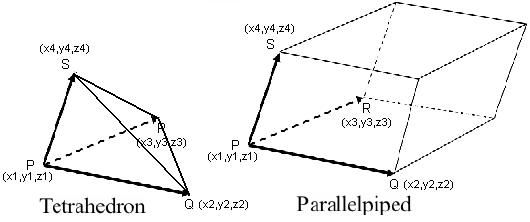# Parellelepiped, Tetrahedron Volume Calculation

Calculates the volumes of parallelepiped and tetrahedron for given vertices. The tetrahedron is a regular pyramid.Enter the vertex P :, , Enter the vertex Q :, , Enter the vertex R :, , Enter the vertex S :, , Volume of Parellelepiped : Volume of Tetrahedron   :
Volume of a Parallelepiped : Geometrically, the absolute value of the triple product represents the volume of the parallelepiped whose edges are the three vectors that meet in the same vertex.
Formula of volume is : $$V=(x4-x1) \times [{(y2-y1) \times (z3-z1)}-{(z2-z1) \times (y3-y1)}];$$ $$+ (y4-y1) \times [{ (z2-z1) \times (x3-x1)}-{(x2-x1) \times (z3-z1)}];$$ $$+ (z4-z1) \times [{ (x2-x1) \times (y3-y1)}-{(y2-y1) \times (x3-x1) }];$$ Volume of a Tetrahedron : The volume of a tetrahedron is equal to 1/6 of the absolute value of the triple product. The tetrahedron has four faces which are equilateral triangles and has 6 edges in regular tetrahedron having equal in length, the regular tetrahedron has four vertices and 3 faces meets at any one of vertex.
The volume of tetrahedron is :
$$\text{Tetrahedron volume} = \frac{ \text{Parallelepiped volume (V)}} {6}$$